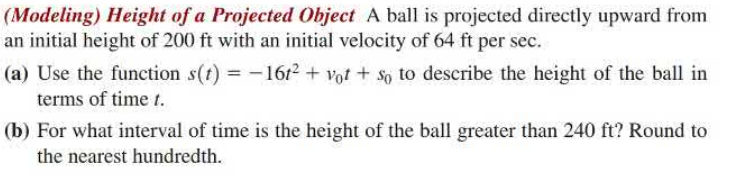### Still have math questions?

Algebra
Question(Modeling) Height of a Projected Object A ball is projected directly upward from an initial height of $$200 ft$$ with an initial velocity of $$64 ft$$ per sec.

(a) Use the function $$s ( t ) = - 16 t ^ { 2 } + v _ { 0 } t + s _ { 0 }$$ to describe the height of the ball in terms of time $$t$$ .

(b) For what interval of time is the height of the ball greater than $$240 ft$$ ? Round to the nearest hundredth.

$$\left. \begin{array} { l } { \text { 2. (a) } s ( t ) = - 16 t ^ { 2 } + 64 t + 200 } \\ { \text { (b) between } 0.78 sec \text { and } 3.22 sec } \end{array} \right.$$